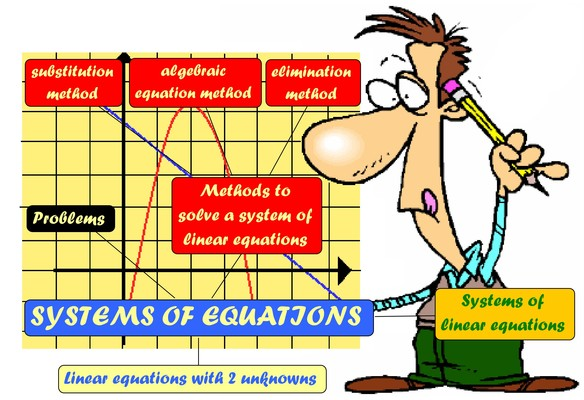Graphing Systems
4 years ago
ksmith146
Save
Edit
Host a game
Live GameLive
Homework
Solo Practice
Practice18 QuestionsShow answers
• Question 1
900 seconds
Q. What is the solution to a system of equations?
The point that the equations have in common.
The point where the two lines intersect.
All of the above.
• Question 2
900 secondsQ. What is the solution to this system?
(1, -1)
(-1, 1)
(0, -2)
(2, 0)
• Question 3
900 secondsQ. What is the solution to this system?
One Solution
No solution
Infinitely Many Solutions
• Question 4
900 seconds
Q. Solve this system of equations by graphing.
y = 2/3x - 2
y = -x + 3
(0,3)
(0,-3)
(3,0)
(-3,0)
• Question 5
900 seconds
Q. Solve this system of equations by graphing.
y = 1/2x + 2
y = -3x + 9
(2,3)
(-2,-3)
(3, 2)
(-3,2)
• Question 6
900 seconds
Q. Solve the system by graphing:
y = 6x - 4
y = -x + 3
(1, 2)
(2, 1)
(4, 5)
(2, 4)
• Question 7
900 secondsQ.

What is the solution?
(2, 2)
(3, 2)
(3, 4)
(2, 3)
• Question 8
900 seconds
Q. If a system of equations has no solution, what does the graph look like?
intersecting lines
parallel lines
same lines
• Question 9
900 secondsQ. Steffen graphed two lines in order to find the solution to a given system of equations.
What is the solution?
(-3,-8)
(-8,-3)
(3,-8)
(8,3)
• Question 10
900 seconds
Q. Solve by graphing.
y = 2x + 1
y = 4x - 1
(1,3)
(-1,-3)
(-1,3)
(3,1)
• Question 11
900 seconds
Q. Solve by graphing:
y = 2x -6
y = 2x +3
(-1.5,-4)
(1.5,4)
No solution (parallel lines)
Infinitely many solutions
• Question 12
900 seconds
Q. Solve by graphing:
y = -4/2x + 3
y = -2x + 3
(0,3)
(3,0)
No solution(parallel lines)
Infinitely many solutions
• Question 13
900 secondsQ. What is the solution?
1
-2
(1, 2)
(1, -2)
• Question 14
900 seconds
Q. The solution (x, y) to a system of equations is the point where they...?
Run off the graph
Don't touch
Intersect
Exist
• Question 15
900 secondsQ. What is the solution to the system?
(0, 3)
(1, -1)
(-3, 1)
(1, 3)
• Question 16
900 secondsQ. What is the solution to the system?
(3, -1)
(2, -6)
No Solution
(6, -2)
• Question 17
900 seconds
Q. Solve the system by graphing:
y = -1/3 x - 4
y = 2x + 3
(-3, -3)
(3, 3)
(3, -3)
(3, 0)
• Question 18
900 seconds
Q. Solve the system by graphing:
y = 3x - 2
y = 4